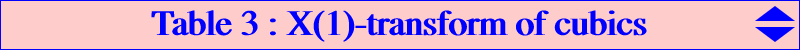The X(1)-transform (or X(1)-isoconjugation) maps the point M(x : y : z) to the point M'(a/x : b/y : c/z). Its fixed points are X(366) and harmonic associates. When K = pK(X6,P), we have K' = pK(X2,Q) where Q has TRILINEAR coordinates the barycentric coordinates of P.P K Q K' X(30) K001 Neuberg cubic ? X(2) K002 Thomson cubic X(75) K034 Spieker perspector cubic X(3) K003 McCay cubic X(63) X(20) K004 Darboux cubic ? X(5) K005 Napoleon cubic ? X(4) K006 Orthocubic cubic X(92) X(384) K020 Brocard 4th cubic X(1965) X(512) K021 Brocard 5th cubic X(661) X(99) K035 Steiner cubic X(799) X(6) K102 Grebe cubic X(1) X(27) K109 X(286) X(385) K128 X(1966) X(69) K169 X(304) X(63) X(69) K007 Lucas cubic ? X(316) K008 Droussent cubic X(92) X(264) K045 Euler perspector cubic X(189) X(309) K133 X(75) X(76) K141 X(48) X(3) K146 X(329) X(322) K154 X(19) X(4) K170